# Python Variable Types

Here you will learn about Python variable types with example. Here Python variable types means Python variable's data type.

Based on variable's data type, python interpreter allocates the memory and decides what can be stored in the reserved memory.

In Python, when you directly initialize an integer value to a Python variable, then that variable can be called as integer type variable at that time, and when you initialize floating-point value to the same variable, then at that time, that variable can be called as floating-point type variable.

## Python Variable Type Example

Here is an example program, uses three variables in python namely m, n, and r

```# Python Variable Types - Example Program
m=54
n=45
r=0
r=m+n
print("sum = ", r)
r=m-n
print("Subtract = ", r)
r=m*n
print("Multiply = ", r)
r=m/n
print("Divide = ", r)
```

Here is the output produced by the above Python variable type example program: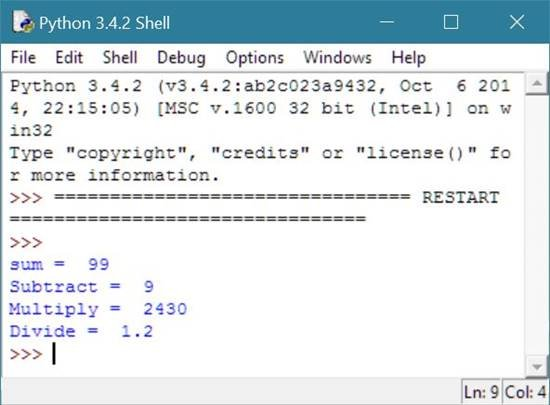As you can see from the above Python variable type program, the same variable, that is, r holds both type of value, that is, integer type value and floating-point type value.

If you want to convert the variable r (floating-point type) into r (integer type) when performed division using m/n, then use int(r) as shown in the given code:

`print("Divide = ", int(r));`

From the above code, int(r) will return the integer value of r

## Use Python Variable Types in Right Way

You must have to concentrate on python variable types, as many python programmer makes mistake in programming with python variable types with wrong handling of python variable types when asking user to enter some details and perform according to user's value.

Let's take an example given below on python variable types.

```# Python Variable Types Example
print("Let's suppose you are a poor man with six family \
in following ways:\n");
house_rent = input("Enter house rent: ");
transport = input("Enter transport rent: ");
school_fee = input("Enter school fee: ");
food = input("Enter food cost: ");
electric_bill = input("Enter electricity bill: ");
other = input("Enter other charges: ");
grand_total = house_rent + transport + school_fee + food + electric_bill + other;
print("Total spending: ", grand_total);```

Here is the sample run of the above example code of python variable types:

This is the initial output or first output: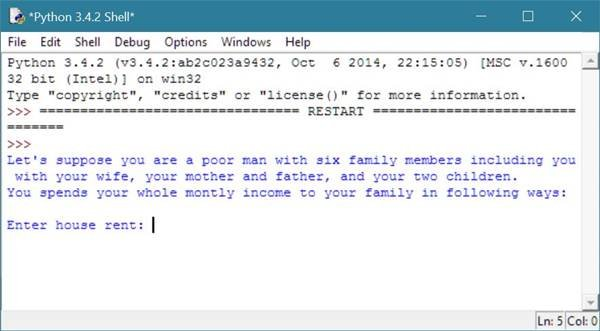Now enter the details as per your spending, here is the sample run. Below is the output after entering all the details: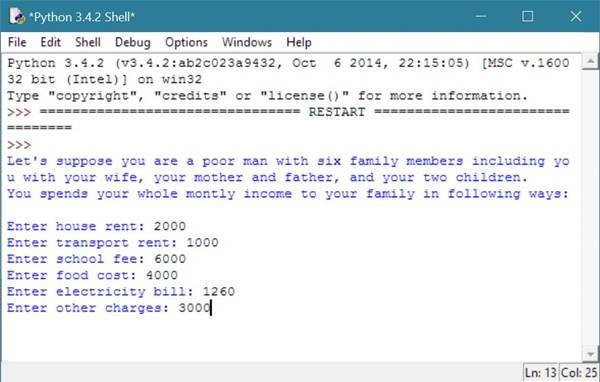After entering the detail, press ENTER key to see the output.

When you will press the enter button, then all the variable will be summed up and the value of the grand_total variable will be printed as output.

Here are the values entered by us:

• Enter house rent: 2000
• Enter transport rent: 1000
• Enter school fee: 6000
• Enter food cost: 4000
• Enter electricity bill: 1260
• Enter other charges: 3000

After the above detail, when you press the ENTER key, the following code will run up.

```grand_total = house_rent + transport + school_fee + food + electric_bill + other;
print("Total spending: ", grand_total);```

The first line will be converted into.

`grand_total = 2000 + 1000 + 6000 + 4000 + 1260 + 3000`

Now grand total will hold the value 200010006000400012603000 as this will not sum as integer, rather this will concatenated as string value. If you want to sum up all the values of the string as integer value, then you must have to type cast the value either directly upon entering the value or later when summing up like:

`house_rent = int(input("Enter house rent: "))`

Or

`int(house_rent)`

as shown in the following example:

```# Python Variable Types Example
print("Let's suppose you are a poor man with six family \
in following ways:\n");
house_rent = int(input("Enter house rent: "));
transport = int(input("Enter transport rent: "));
school_fee = int(input("Enter school fee: "));
food = int(input("Enter food cost: "));
electric_bill = int(input("Enter electricity bill: "));
other = int(input("Enter other charges: "));
grand_total = house_rent + transport + school_fee + food + electric_bill + other;
print("Total spending: ", grand_total);```

Or

```# Python Variable Types Example
print("Let's suppose you are a poor man with six family \
in following ways:\n");
house_rent = input("Enter house rent: ");
transport = input("Enter transport rent: ");
school_fee = input("Enter school fee: ");
food = input("Enter food cost: ");
electric_bill = input("Enter electricity bill: ");
other = input("Enter other charges: ");
grand_total = int(house_rent) + int(transport) + int(school_fee) + int(food) + int(electric_bill) + int(other);
print("Total spending: ", grand_total);```

You will see the same output given below, produced by both the above example code of variable types in Python.As you can see from the above output, this time, you will successfully generated the addition of all the spending you have done in a month on your family.

You don't have to worry about direct initialized or assigned value to python variable as at that time, variable automatically become the type of value you are initializing as given in the following updated program of the above one.

```# Python Variable Types Example
print("A poor man has six family members including \
himself with his wife, his mother and father, \
and his two children.");
print("He spends his whole montly income to his family.");
house_rent = 2000;
transport = 1000;
school_fee = 6000;
food = 4000;
electric_bill = 1260;
other = 3000;
total = house_rent + transport + school_fee + food + electric_bill + other;
print("\nTotal spending of the man in a month =",total);```

Here is the sample output produced by the above python variable type example code: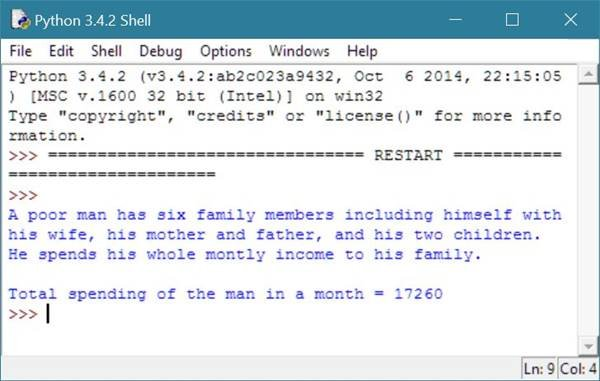## How to Determine Variable's Type in Python ?

You can use the function type() available in Python, to determine the type of variable in Python.

Here is an example showing the use of type() function in determining variable's type in Python:

```# How to determine variable's type in Python
i = 10;
f = 10.45;
b = True;
strng = "Python Variable Type";
print("If i contains '10', then i is of",type(i),"type variable in Python.");
print("If f contains '10.45', then f is of",type(f),"type variable in Python.");
print("If b contains 'True', then b is of",type(b),"type variable in Python.");
print("If strng contains 'Python Variable Type', then str is of",type(strng),"type variable in Python.");```

Here is the sample output of the above finding variable type program in python: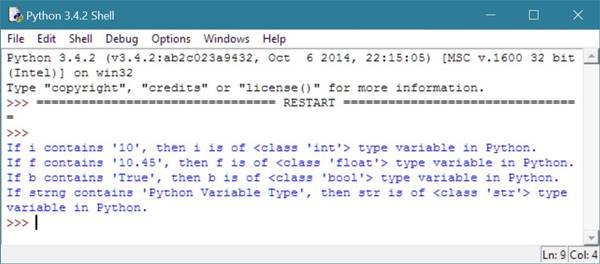Tools
Calculator